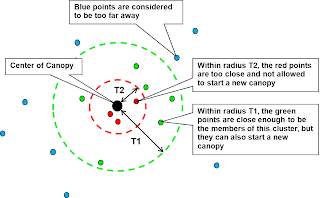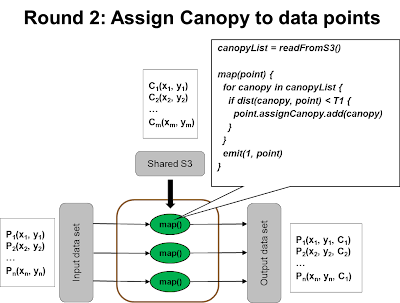## Thursday, April 21, 2011

### K-Means Clustering in Map Reduce

Unsupervised machine learning has broad application in many e-commerce sites and one common usage is to find clusters of consumers with common behaviors. In clustering methods, K-means is the most basic and also efficient one.

K-Means clustering involve the following logical steps

1) Determine the value of k
2) Determine the initial k centroids
3) Repeat until converge
- Determine membership: Assign each point to the closest centroid
- Update centroid position: Compute new centroid position from assigned members

Determine the value of K
This is basically asking the question of: "How many clusters you are interested to discover ?"
So the answer is specific to the problem domain.

One way is to try different K. At some point, we'll see increasing K doesn't help much to improve the overall quality of clustering. Then that is the right value of K.

Notice that the overall quality of cluster is the average distance from each data point to its associated cluster.

Determine the initial K centroids
We need to pick K centroids to start the algorithm. So one way to pick them is to randomly pick K points from the whole data set.

However, picking a good set of centroids can reduce the number of subsequent iterations and by "good" I mean the K centroid should be as far apart to each other as possible, or even better the initial K centroid is close to the final K centroid. As you can see, choosing the random K points is reasonable but non-optimum.

Another approach is to take a small random sample set from the input data set and do a hierarchical clustering within this smaller set (note that hierarchical clustering is not-scaling to large data set).

We can also partition the space into overlapping region using canopy cluster technique (describe below) and pick the center of each canopy as the initial centroid.

Iteration
Each iteration is implemented as a Map/Reduce job. First of all, we need a control program on the client side to initialize the centroid positions, kickoff the iteration of Map/Reduce jobs and determine whether the iteration should end ...

``````kmeans(data) {
initial_centroids = pick(k, data)
writeToS3(initial_centroids)
old_centroids = initial_centroids
while (true){
map_reduce()
if change(new_centroids, old_centroids) < delta {
break
} else {
old_centroids = new_centroids
}
}
return result
}``````

Within each iteration, most of the processing will be done in the Map task, which determine the membership for each point, as well as compute a partial sum of each member points of each cluster.

The reducer did the easy job by aggregating all partial sums and compute the update centroid position, and then out them into a shared store (S3 in this case) that can be picked up by the Map/Reduce job of next round.Complexity Analysis
Most of the work is done by the Mapper and the workload is pretty balanced. So the time complexity will be O(k*n/p) where k is number of clusters, n is number of data points and p is number of machines. Note that the factor of k comes in at the closest_centroid() function above when comparing each data point with each intermediate centroid as follows ...
``````closest_centroid(point, listOfCentroids) {
bestCentroid = listOfCentroids
minDistance = INFINITY
for each centroid in listOfCentroids {
distance = dist(point, centroid)
if distance < minDistance {
minDistance = distance
bestCentroid = centroid
}
}
return bestCentroid
}``````

If we partition the space into proximity regions, we only need to compare each point with centroid within the same proximity region and treat other centroids infinite distance. In other words, we don't have to compare each point with all k centroids.

Canopy clustering provide such a partitioning mechanism.

Canopy Clustering
To define the proximity region (canopy), we can draw a circle (or hypersphere) centered at a data point. Points outside this sphere is considered to be too far.

However, if we apply this definition to every point, then we will have as many proximity region as the number of points, which ends up doesn't save much processing. We also observed that points are very close by each other can stay in the same region without each point creating their own. Therefore, we can draw a smaller circle within the big circle (with the same center) such that data points within the small circle is not allowed to form its own proximity region.Notice that each proximity region can overlap with each other and the degree of overlapping will be affected by the choice of T1. Also the choice of T2 affects how many canopies will be formed. Picking the right number of T1 and T2 is domain-specific, and also depends on the number of clusters and the space volume. If there is a small number of clusters within a big space, then a bigger T1 should be chosen.

To create the canopies (and mark the data points with the canopies), we will do the following steps ...
1) Create the canopy centers, with one scan
• Keep a list of canopies, initially an empty list
• Scan each data point, if it is within T2 distance of existing canopies, discard it. Otherwise, add this point into the list of canopies2) Assign data points to the canopies, with another scan
• Scan each data point, if it is within T1 of the canopyA, add A as the assigned canopy to the data point. Notice that the data point can be assigned to multiple canopies
• When done, each data point will look likeNotice that now the input data points has been added with an extra attribute that contains the assigned canopies. When compare the point with the intermediate centroids, we only need to compare centroids within the same canopy. Here is the modified version of the algorithm ...

``````closest_centroid(point, listOfCentroids) {
bestCentroid = listOfCentroids
minDistance = INFINITY
for each cent in listOfCentroids {
if (not point.myCanopy.intersects(cent.myCanopy)) {
continue
}
distance = dist(point, centroid)
if distance < minDistance {
minDistance = distance
bestCentroid = centroid
}
}
return bestCentroid
}``````